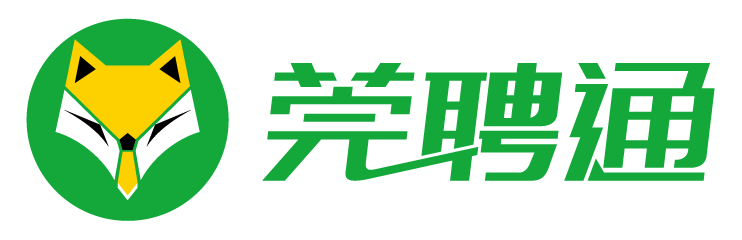• 登录 | 注册• 不限
• 市场销售
• 行政人事
• 文职文员
• 城建装修
• 客户服务
• 会计财务
• 普工技工
• 文体培训
• 设计包装
• 百货零售
• 工厂工业
• 餐饮休闲
• 金融保险
• 管理运营
• 物流贸易
• 司机后勤
• 网络硬件
• 机械仪表
• 咨询顾问
• 电子通讯
• 医疗美容
• 翻译法律
• 轻工工艺
• 化工制药
• 摄影影视
• 能源环保
• 编辑发行
• 其他分类
• 产品工艺

• 不限
• 客户服务经理
• 客户数据库管理
• 客户关系管理
• 客户培训
• 客户咨询
• 热线咨询
• 客户服务
• 售前/售后支持
• 投诉处理
• 客户主任
• 接待处主任
• 客户服务其他相关职位

• 东城
• 南城
• 松山湖
• 大朗
• 寮步
• 莞城
• 茶山
• 大岭山
• 石碣
• 中堂
• 万江
• 虎门
• 石龙
• 高埗
• 厚街
• 长安
• 沙田
• 樟木头
• 麻涌
• 道滘
• 凤岗
• 塘厦
• 清溪
• 黄江
• 常平
• 东坑
• 谢岗
• 桥头
• 横沥
• 企石
• 石排
• 望牛墩
• 洪梅

• 不限
• 今天
• 三天内
• 一周内
• 一个月内
• 三个月内

• 不限
• 全职
• 兼职

• 不限
• 1000元以下
• 1000-2000元
• 2000-3000元
• 3000-5000元
• 5000-8000元
• 8000-1.2万元
• 1.2万-2万元
• 2万元以上
• 面议